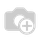# [odoo-9] What are the new python library used in core addons?

Hello All,

What are the new library used in core addons module in v9.

Anybody has idea can provide list of new libraries/packages used.

Thanks & Rgds,

AnilHi Anil,

You can find all required libraries on Github. Which you can see here.The main new used one is lessc.
At the moment of writing this answer:

```Babel==1.3
Jinja2==2.7.3
Mako==1.0.1
MarkupSafe==0.23
Pillow==2.7.0
Python-Chart==1.39
PyYAML==3.11
Werkzeug==0.9.6
argparse==1.2.1
decorator==3.4.0
docutils==0.12
feedparser==5.1.3
gdata==2.0.18
gevent==1.0.1
greenlet==0.4.5
jcconv==0.2.3
lxml==3.4.1
mock==1.0.1
ofxparse==0.14
passlib==1.6.2
psutil==2.2.0
psycogreen==1.0
psycopg2==2.5.4
pyPdf==1.13
pydot==1.0.2
pyparsing==2.0.3
pyserial==2.7
python-dateutil==2.4.0python-ldap==2.4.19
python-openid==2.2.5
pytz==2014.10
pyusb==1.0.0b2
qrcode==5.1
reportlab==3.1.44
requests==2.6.0
simplejson==3.6.5
six==1.9.0
suds-jurko==0.6
unittest2==0.8.0
vatnumber==1.2
vobject==0.6.6
wsgiref==0.1.2
xlwt==0.7.5```

Yenthe

1 Comment